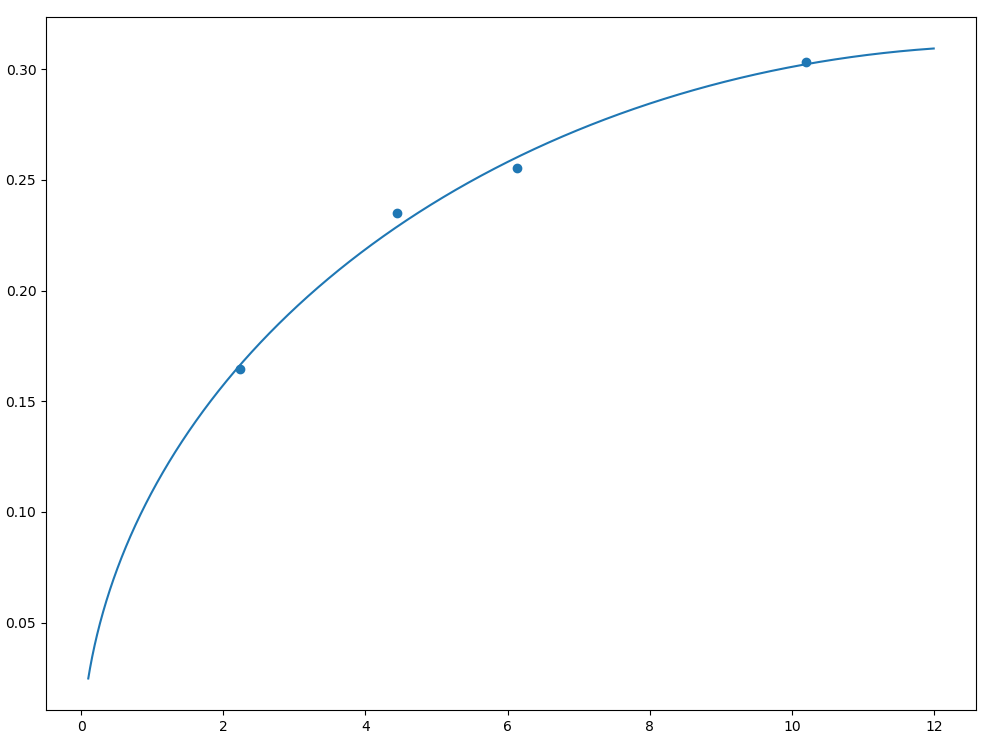• python 拟合 leastsq 最小二乘python 拟合 leastsq 最小二乘 python 拟合 leastsq 最小二乘 scipy库提供了函数leastsq函数用于非常规函数的参数拟合 代码实现如下： import numpy as np from scipy.optimize import ...
python 拟合 leastsq 最小二乘python 拟合 leastsq 最小二乘
python 拟合 leastsq 最小二乘
scipy库提供了函数leastsq函数用于非常规函数的参数拟合
代码实现如下：
import numpy as np
from scipy.optimize import leastsq

#dainiheshuju
data_x = np.array([2.22867,4.44599,10.20322,6.13807])
data_y = np.array([0.16449,0.23483,0.3031,0.25519])

def reso_mcnp(E,p0,p1,c):
return p0 + np.sqrt(p1*(E+c*E*E))

def error(p,x,y):
p0,p1,c = p
return reso_mcnp(x,p0,p1,c)-y

pp = [1,1,1]
Para = leastsq(error,pp,args=(data_x,data_fwhm))

p0,p1,c = Para

print(Para)

Y_reso = reso_mcnp(X_E,p0,p1,c)
plt.figure(figsize=(12,9))
plt.scatter(data_x,data_fwhm)
plt.plot(X_E,Y_reso)
plt.show()


拟合获取了p0、p1、c三个参数，打印参数如下：
(array([-0.01536602,  0.01614392, -0.03796763]), 1)

拟合效果如图展开全文数据分析
• 以前都是用origin来进行拟合，但是参数...花了一下午了解了一下用Python拟合已有的函数，感觉还不错，分享出来给大家。初学Python，大神们多多包涵。# -*- coding: utf-8 -*-"""Created on Tue Nov 24 14:50:56 20...
以前都是用origin来进行拟合，但是参数初值需要猜测，有时候不一定能够得到正确结果。后来用过MATLAB的工具箱，可以拟合，但电脑要安装MATLAB还是占挺大空间的。花了一下午了解了一下用Python来拟合已有的函数，感觉还不错，分享出来给大家。初学Python，大神们多多包涵。# -*- coding: utf-8 -*-"""Created on Tue Nov 24 14:50:56 2020@author: fan"""import pandas as pdimport numpy as npimport matplotlib.pyplot as pltfrom scipy.optimize import curve_fitdf1 = pd.read_excel('F:/langmuir.xls',header=None,usecols="A") #导入数据，A列为x轴备用df2 = pd.read_excel('F:/langmuir.xls',header=None,usecols="B") #导入数据，B列为y轴备用Ce0 = np.array(df1) #dataframe转数组，此时为单列数组，需要转置为单行Ce = Ce0[:,0]  #转置print(Ce)qe0 = np.array(df2) #dataframe转数组，此时为单列数组，需要转置为单行qe = qe0[:,0] #转置print(qe)# 这里的函数可以自定义任意形式。def func(Ce, qm, Ka):return qm*Ka*Ce/(1+Ka*Ce)# popt返回的是给定模型的最优参数。我们可以使用pcov的值检测拟合的质量，其对角线元素值代表着每个参数的方差。param_bounds=([0,0],[np.inf,np.inf]) #参数上下限，第一个方括号为所有参数下限，第二个为所有参数上限popt, pcov = curve_fit(func, Ce, qe, bounds=param_bounds) #拟合函数qm = poptKa = poptqe_pre = func(Ce,qm,Ka) #拟合y值plot1 = plt.plot(Ce, qe, 's',label='original values')plot2 = plt.plot(Ce, qe_pre, 'r',label='polyfit values')plt.xlabel('Ce')plt.ylabel('qe')plt.legend(loc=4) #右下角画图例SSE = ((qe_pre-qe)**2).sum()chi_square = ((qe-qe_pre)**2/qe_pre).sum()#计算R平方from sklearn.metrics import r2_scorer2 = r2_score(qe, qe_pre)print('qm=',qm)print('Ka=',Ka)print('拟合R方为:',r2)print('SSE =',SSE)print('chi_square =',chi_square)
展开全文• 我正试图为装箱设计一个最适合的算法。装箱问题：在给定容量的情况下，尽量减少用于包装物品的箱子数量。在这个启发应该做的是尽量将物品放在能容纳物品的最满的...我在Python中尝试了以下方法：item = ['a', 'b'...
我正试图为装箱设计一个最适合的算法。装箱问题：在给定容量的情况下，尽量减少用于包装物品的箱子数量。在这个启发应该做的是尽量将物品放在能容纳物品的最满的箱子里，也就是说，能留下最少空间的箱子如果找不到箱子，启动一个新箱子。为了写这个，我做了一个辅助卷。对于要放置的项目，将体积添加到每个箱子中。容量最大的箱子(这仍然可行)将得到该物品。我在Python中尝试了以下方法：item = ['a', 'b', 'c', 'd', 'e']volume = [9,5,6,7,2]V = 15class Bin(object):def __init__(self):self.items = []self.sumVolume = 0self.auxvolumeSpace = 0def add(self, item, volume):self.items.append(item)self.sumVolume += volumeself.auxvolumeSpace = self.sumVolumedef __str__(self):# Printable representationreturn 'Bin(TotalVolume=%d, products=%s)' % (self.sumVolume, str(self.items))if __name__ == '__main__':def packAndShow(volume, maxVolume):bins = pack(volume, maxVolume)print('Solution using', len(bins), 'bins:')for i in bins:print(i)print('The total amount of bins needed, using the Best fit Algorithm is: ',len(bins))def auxiliaryspace(volume, maxVolume):bins = []maxvolumespace = -1for bin in bins:if bin.sumVolume + volume <= maxVolume:bin.auxvolumeSpace += volumeif bin.auxvolumeSpace >= maxvolumespace:maxvolumespace = bin.auxvolumeSpacereturn maxvolumespacedef pack(volume, maxVolume):bins = []for i in range(len(volume)):mv = auxiliaryspace(volume[i], maxVolume)for bin in bins:if mv > 0 and bin.auxvolumeSpace == mv:bin.add(item[i], volume[i])breakelse:bin = Bin()bin.add(item[i], volume[i])bins.append(bin)return binspackAndShow(volume, V)问题是所有的东西都放在一个新的箱子里。结果如下：^{pr2}\$我认为问题出在“辅助空间”部分。我认为返回的值(我想要的)不正确。。有人能帮忙吗？在
展开全文• python拟合对数正态分布使用的是scipy.stats.lognorm这个包，这个包的使用看官方文档就行，但是其中有一个很迷的地方，网上也有人提到了这个很迷的地方：关于scipy对数正态分布的误区，然后Stack Overflow里也有人...
用python拟合对数正态分布使用的是scipy.stats.lognorm这个包，这个包的使用看官方文档就行，但是其中有一个很迷的地方，网上也有人提到了这个很迷的地方：关于scipy对数正态分布的误区，然后Stack Overflow里也有人给出了解释Stack Overflow大佬的解释说明，，其实Stack Overflow和官网都有解释，可能是我的英语还是太差了吧，导致始终觉得需要看好久才能理解，所以这里来记录下这个漏洞以及我给出的例子。官网链接还是必须的，不管其他人写的多清楚，看下官网总没错。
Stack Overflow中的解释说明：1. 参数的解释
下图是官网的说明，首先对数正态分布的概率密度函数就看下面的就行了。要调用这个包的概率密度函数的参数主要是有如下几个lognorm.pdf(x, s, loc, scale)，下面就说明下各个参数的含义：
x也就是自变量，是一个list或series啥的都可以，返回的是每个x处的对数正态分布的概率密度函数。s是形状参数，loc是一个参数，你的数据都会被加上loc，以便使得0成为数据范围的下确界，对方差没有影响。因为对数正态分布嘛，取了对数的，一般都是(0,无穷)。scale类似于标准差，但不是。听我下面的说明。我们假设一个一维的数组x，这个一维的数组x的分布是对数正态分布。那么要得到这个一维数组x的概率密度函数就可以使用lognorm.pdf(x, s, loc, scale)，其中的x可以输入该数组，也可以输入一个自己设置的，比如x1=np.linspace(0,3,200)，因为这个是参数估计，所以只要得到参数值，其实就已经确定分布了，这个x就没用了，任何一个一维数组都可以给出对应位置的概率密度值。那么如何根据x得到lognorm.pdf(x, s, loc, scale)中的参数呢？下面一个一个参数来说明：

s：s=np.std(np.log(x))，也就是先对x求log，得到正态分布的数组，然后对这个正态分布的数组求标准差，这个标准差就是s。
loc设置为0就可以，用默认值就行，不用去设置。如果源数据有偏移可以设置下loc。这个参数我简单尝试试验了下，但不确定，若有问题，大佬可以指正，谢谢。
scale：scale=math.exp(np.mean(np.log(x)))，也就是说先对x求log，得到正态分布的一维数组，然后对正态分布的x求均值，然后exp（得到的均值）就是scale了。
这里贴上Stack Overflow上的一个例子。

import math
from scipy import stats

# standard deviation of normal distribution
sigma = 0.859455801705594
# mean of normal distribution
mu = 0.418749176686875
# hopefully, total is the value where you need the cdf
total = 37

frozen_lognorm = stats.lognorm(s=sigma, scale=math.exp(mu))
frozen_lognorm.cdf(total) # use whatever function and value you need here

下面有个老兄也吐槽scipy的这个参数非人类，哈哈，贴上给大家一乐。2. 我写的例子，证明上述说明
为了方便我就放图了，反正也很简单，大家看看就完了，我感觉也不必去自己跑了。代码如下：
# 生成正态分布的一维数组
import numpy as np
ser1 = np.random.normal(1, 2, 10000)
print(np.mean(ser1), " ", np.var(ser1))

#生成对数正态分布的一群点
ser2 = np.exp(ser1)
print(ser2)

print(ser1)

print("符合对数正态分布一维数组的均值和标准差",np.mean(ser2),np.var(ser2))

# 验证对数正态分布的均值和方差
mu = math.exp(1+2)
sig2 = (math.exp(4)-1)*math.exp(2*1+4)
print("均值{0},方差{1}".format(mu , sig2))
### 验证成功，方差因为比较大，有一定差异，但是相对误差还是不大的。

### 使用lognorm这个函数取输出理论的均值和标准差，
### 可以看到和我们计算出的理论值完全一样。
### 这既证明理论值是正确的，也证明我们对这个函数的参数的说明是正确的。
mu = lognorm.mean( s=2 , scale=math.exp(1))
sig2 = lognorm.var( s=2 , scale=math.exp(1))
print("均值{0},方差{1}".format(mu , sig2))

# 绘制对数正态分布概率密度函数图形
x=np.linspace(0,50,20000)
plt.plot(x,lognorm.pdf(x=x , s=2 , scale=math.exp(1)))
plt.show()

# 对loc这个参数进行一些试验，以简单了解loc这个参数的含义
mu = lognorm.mean( s=2 , loc = 4,scale=math.exp(1))
sig2 = lognorm.var( s=2 ,loc =  4, scale=math.exp(1))
print("均值{0},方差{1}".format(mu , sig2))

# 对loc这个参数进行一些试验，以简单了解loc这个参数的含义
mu = lognorm.mean( s=2 , loc = -4,scale=math.exp(1))
sig2 = lognorm.var( s=2 ,loc =  -4, scale=math.exp(1))
print("均值{0},方差{1}".format(mu , sig2))

mu = lognorm.mean( s=2 , scale=math.exp(1))
sig2 = lognorm.var( s=2 , scale=math.exp(1))
print("均值{0},方差{1}".format(mu , sig2))


结束啦


展开全文scipy 对数正态分布
• 1.多项式拟合范例：import matplotlib.pyplot as pltimport numpy as np#潘海东,2014/1/13x = np.arange(1, 17, 1)y = np.array([4.00, 6.40, 8.00, 8.80, 9.22, 9.50, 9.70, 9.86,10.00, 10.20, 10.32, 10.42, ...
• I would like to fit data with integral function(truncated gamma distribution).I tried following code, but errors occur. I am appreciate if you would kind help me. Thank you very much in advance.%matpl...
• 在很多情况下，大家的工作会遇到y数据与x数据存在相关性，但无法知道y与x是那种相关，需要具体知道两者关系，我们可以用拟合模型来完成这个事情。1：随机源数据很多初学者想动手尝试的时候，苦于无源数据，就此放弃...
• /usr/bin/python # -*- coding:utf-8 -*- # @Author: DingQinbin # @Time : 2020/10/20 14:11 import pylab import numpy as np import sys, os from scipy.optimize import curve_fit def func(x, a, b, c): ...
• 要用PID对小车速度进行控制，需要求得pwm-enc之间的增益系数Ka，于是我采集了多组pwm-enc样本数据，用直线拟合的方法求Ka，具体python代码如下：import matplotlib.pyplot as plt import math def linefit(x...
• 这篇文章主要介绍了python matplotlib拟合直线的实现,文中通过示例代码介绍的非常详细，对大家的学习或者工作具有一定的参考学习价值,需要的朋友可以参考下代码如下import numpy as npimport matplotlib.pyplot as ...
• 研究生数值分析课程_最全python拟合程序_python（最小二乘法，龙贝格）。自己做的，南大的在读研究生。
• 我们也可以尝试用python编写脚本来拟合数据，方便数据自动化处理。曲线拟合分为一般多项式拟合和指定函数拟合。准备工作：1.建立python环境（https://www.jianshu.com/p/daf5d33ae62e）2.安装模块numpy、matplotlib...
• 找了好久python拟合曲面的方法，没找到，就借鉴 https://blog.csdn.net/Haipai1998/article/details/85345823 这篇博客，对方法进行封装更便于使用。 代码 处理符号问题 def fun(x): round(x, 2) if x >= 0: ...
• 本文实例讲述了Python线性拟合实现函数与用法。分享给大家供大家参考，具体如下：1. 参考别人写的：#-*- coding:utf-8 -*-import mathimport matplotlib.pyplot as pltdef linefit(x , y):N = float(len(x))sx,sy,...
• # Python拟合数据样本的分布# 安装fitter# pip install fitter# 生成一段模拟数据from scipy import statsimport numpy as np# N(0,2)+N(0,10)data1 = list(stats.norm.rvs(loc=0, scale=2, size=70000))data2 = ...
• 大家好,我正在尝试使用python拟合点的曲线,但是我还没有成功,我是使用python的初学者,我发现它没有帮助我.我有一组数据,我想分析哪条线最能描述它(不同阶的多项式).在numpy中,对于多项式拟合,有polyfit()和polyval()...
• 例子：拟合一种函数Func，此处为一个指数函数。出处：SciPy v1.1.0 Reference Guide​docs.scipy.org#Headerimport numpy as npimport matplotlib.pyplot as pltfrom scipy.optimize import curve_fit#Def...
• 这里是一个图形化Python拟合器，其中包含您的方程式和从散点图中提取的数据，您应该使用实际数据进行重新拟合。import numpy, scipy, matplotlibimport matplotlib.pyplot as pltfrom scipy.optimize import curve_...
• python的scipy.optimize包里的curve_fit函数来拟合曲线，当然还可以拟合很多类型的曲线。scipy.optimize提供了函数最小值(标量或多维)、曲线拟合和寻找等式的根的有用算法。import numpy as npimport matplotlib...
• 原博文2019-06-02 14:35 −需要对数据... python的scipy.optimize包里的curve_fit函数来拟合曲线，当然还可以拟合很多类型的曲线。scipy.optimize提供了函数最小值(标量或多维)、...023537相关推荐2019-12-09 15:04...
• python的scipy.optimize包里的curve_fit函数来拟合曲线，当然还可以拟合很多类型的曲线。scipy.optimize提供了函数最小值(标量或多维)、曲线拟合和寻找等式的根的有用算法。import numpy as npimport matplotlib...
• 最小二乘Python 二次拟合 随机生成数据，并且加上噪声干扰构造需要拟合的函数形式，使用最小二乘法进行拟合输出拟合后的参数将拟合后的函数与原始数据绘图后进行对比import numpy as npimport matplotlib.pyplot as ...
• 我想用-a*sin(b*x + c)(或者也可以是-a*sin(2*x))形式的函数来拟合下面所附的数据，a b c作为要确定的值。我使用了scipy.optimize.curve_fit，但它的工作效果不太好(正如您在image中看到的那样)。我该如何改进？在...
• RANSAC算法详解(附Python拟合曲线和平面) 下文博主整理的很详细，请参考： https://lixin97.com/2019/04/10/RANSAC/随机采样一致
• 本文实例为大家分享了Python曲线拟合的最小二乘法，供大家参考，具体内容如下模块导入import numpy as npimport gaosi as gs代码"""本函数通过创建增广矩阵，并调用高斯列主元消去法模块进行求解。"""import numpy ...
• 1 ,2 ,3 ,4 ,5 ,6] y=[ 2.5 ,3.51 ,4.45 ,5.52 ,6.47 ,7.51] a,b,r=linefit(x,y) print("X=",x) print("Y=",y) print("拟合结果: y = %10.5f x + %10.5f , r=%10.5f" % (a,b,r) ) plt.plot(x, y, "r:", linewidth=2)...
• 拟合函数 y = alpha * pow(x, beta)输入： x数组，y数组输出： alpha，beta，相关系数R2from scipy.optimize import leastsqfrom pylab import *import numpy as npxdata = np.array([4.79616, 11.63, 37.5534, ......

# python拟合python 订阅